JavaScript 中 this 指向详细分析(译)神秘的this

this 关键词已经折磨我很久很久了。

• 方法调用(method invocationconsole.log('Hello World!')
• 构造函数调用(constructor invocationnew RegExp('\\d')1. 函数直接调用(function invocation)

function hello(name) {
return 'Hello ' + name + '!';
}
// Function invocation
const message = hello('World');

hello('World') 就是一个函数直接调用：hello 可以解析成一个函数对象，紧跟着是用括号括起来的 World 参数。

// IIFE
const message = (function(name) {
return 'Hello ' + name + '!';
})('World');

1.1 函数直接调用中的 this 指向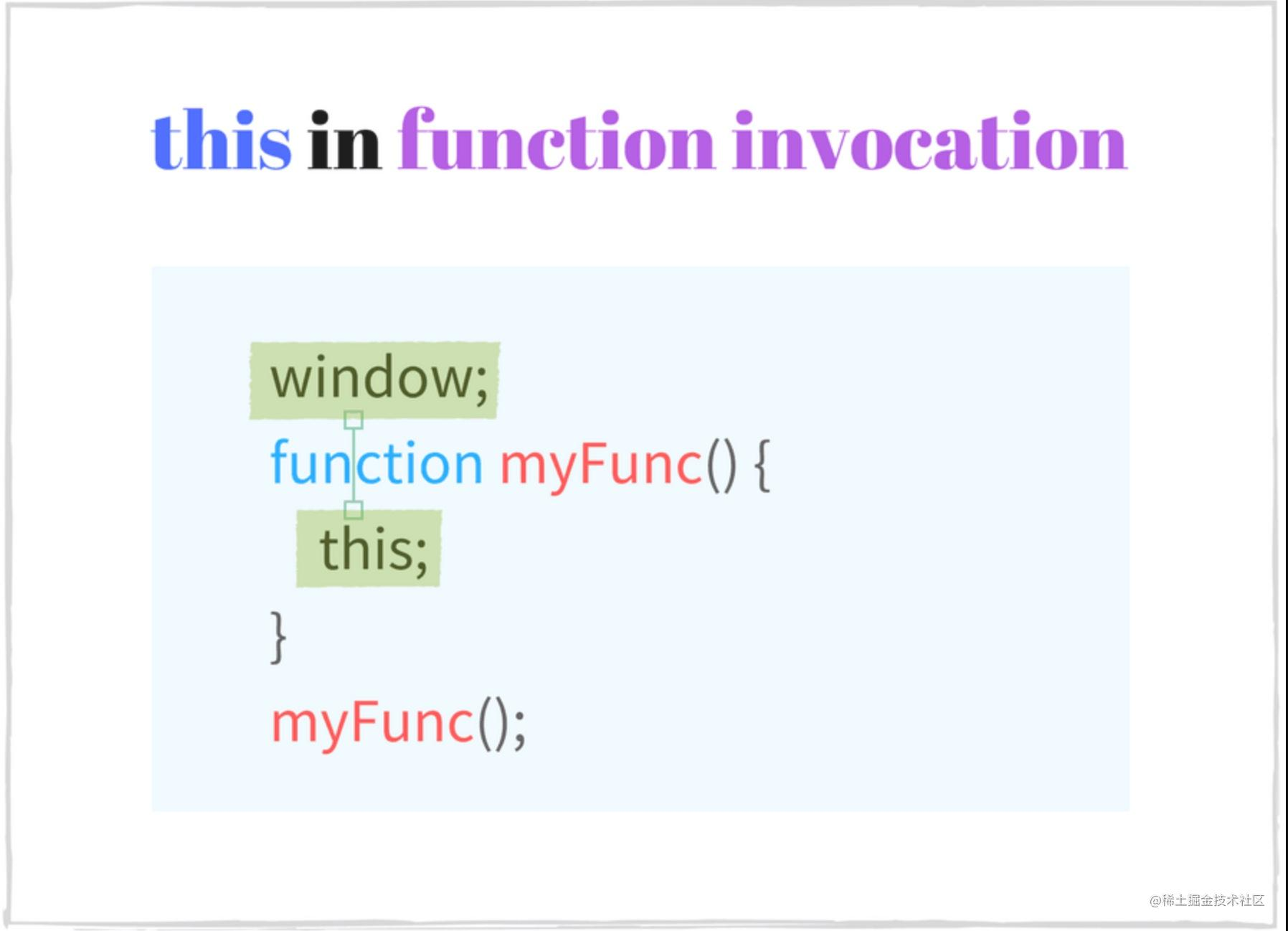function sum(a, b) {
console.log(this === window); // => true
this.myNumber = 20; // add 'myNumber' property to global object
return a + b;
}
// sum() is invoked as a function
// this in sum() is a global object (window)
sum(15, 16);     // => 31
window.myNumber; // => 20

this 在函数外的顶级作用域中使用的时候，同样也是指向全局对象的：

console.log(this === window); // => true
this.myString = 'Hello World!';
console.log(window.myString); // => 'Hello World!'

html 中的 script 中也是一样的。

<!-- In an html file -->
<script type="text/javascript">
console.log(this === window); // => true
</script>

1.2 函数直接调用中的 this 指向（严格模式）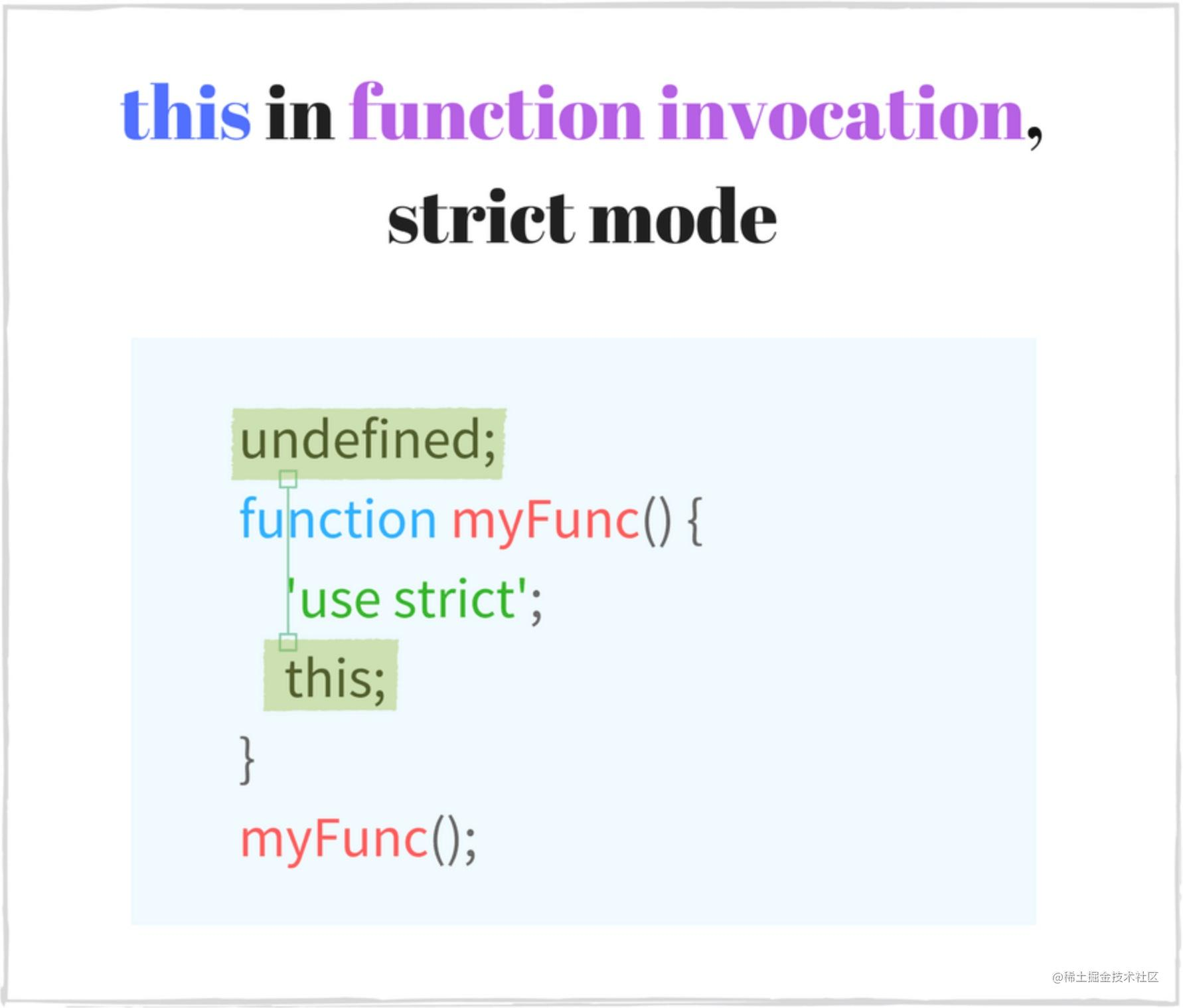function multiply(a, b) {
'use strict'; // enable the strict mode
console.log(this === undefined); // => true
return a * b;
}
// multiply() function invocation with strict mode enabled
// this in multiply() is undefined
multiply(2, 5); // => 10

function execute() {
'use strict';
function concat(str1, str2) {
// the strict mode is enabled too
console.log(this === undefined); // => true
return str1 + str2;
}
console.log(this === undefined); // => true
// concat() is invoked as a function in strict mode
// this in concat() is undefined
concat('Hello', ' World!'); // => "Hello World!"
}
execute();

function nonStrictSum(a, b) {
// non-strict mode
console.log(this === window); // => true
return a + b;
}
function strictSum(a, b) {
'use strict';
// strict mode is enabled
console.log(this === undefined); // => true
return a + b;
}
// nonStrictSum() is invoked as a function in non-strict mode
// this in nonStrictSum() is the window object
nonStrictSum(5, 6); // => 11
// strictSum() is invoked as a function in strict mode
// this in strictSum() is undefined
strictSum(8, 12); // => 20

1.3 陷阱：内部函数的 this 指向

⚠️一个很普遍的陷阱：对于函数直接调用，可能会认为如果是函数内部定义的函数，它的 this 会指向外部函数的上下文。

👍要始终记得，内部函数的 this 指向（除了箭头函数）只和它的调用类型相关（也就是本文介绍的几种类型），而不是它所在函数的上下文。

const numbers = {
numberA: 5,
numberB: 10,
sum: function() {
console.log(this === numbers); // => true
function calculate() {
// this is window or undefined in strict mode
console.log(this === numbers); // => false
return this.numberA + this.numberB;
}
return calculate();
}
};
numbers.sum(); // => NaN or throws TypeError in strict mode

⚠️ numbers.sum() 属于对象的一个方法调用，下一小节会讲。从代码分析，这里 calculate() 的结果其实就是 numbers.sum() 的结果 。因为 calculate 函数定义在 sum 中，sumnumbers 对象的属性，因此当调用 numbers.sum()， 我们会觉得 calculate 中的 this 也指向 numbers 对象。

👍 为了解决这个问题，calculate() 函数必须拥有和 numbers.sum() 相同的 this 指向，这样在 calculate 中才能访问到 this.numberAthis.numberB

const numbers = {
numberA: 5,
numberB: 10,
sum: function() {
console.log(this === numbers); // => true
function calculate() {
console.log(this === numbers); // => true
return this.numberA + this.numberB;
}
// use .call() method to modify the context
return calculate.call(this);
}
};
numbers.sum(); // => 15

calculate.call(this) 会正常执行 calculate()函数，同时会将它的执行上下文变为所传入的第一个参数。

const numbers = {
numberA: 5,
numberB: 10,
sum: function() {
console.log(this === numbers); // => true
const calculate = () => {
console.log(this === numbers); // => true
return this.numberA + this.numberB;
}
return calculate();
}
};
numbers.sum(); // => 15

2 方法调用

const myObject = {
// helloMethod is a method
helloMethod: function() {
return 'Hello World!';
}
};
const message = myObject.helloMethod();

helloMethodmyObject 的一个方法。使用属性访问器 myObject.helloMethod 可以调用这个方法。

const words = ['Hello', 'World'];
words.join(', ');   // method invocation
const obj = {
myMethod() {
return new Date().toString();
}
};
obj.myMethod();     // method invocation
const func = obj.myMethod;
func();             // function invocation
parseFloat('16.6'); // function invocation
isNaN(0);           // function invocation

2.1 方法调用中的 this 指向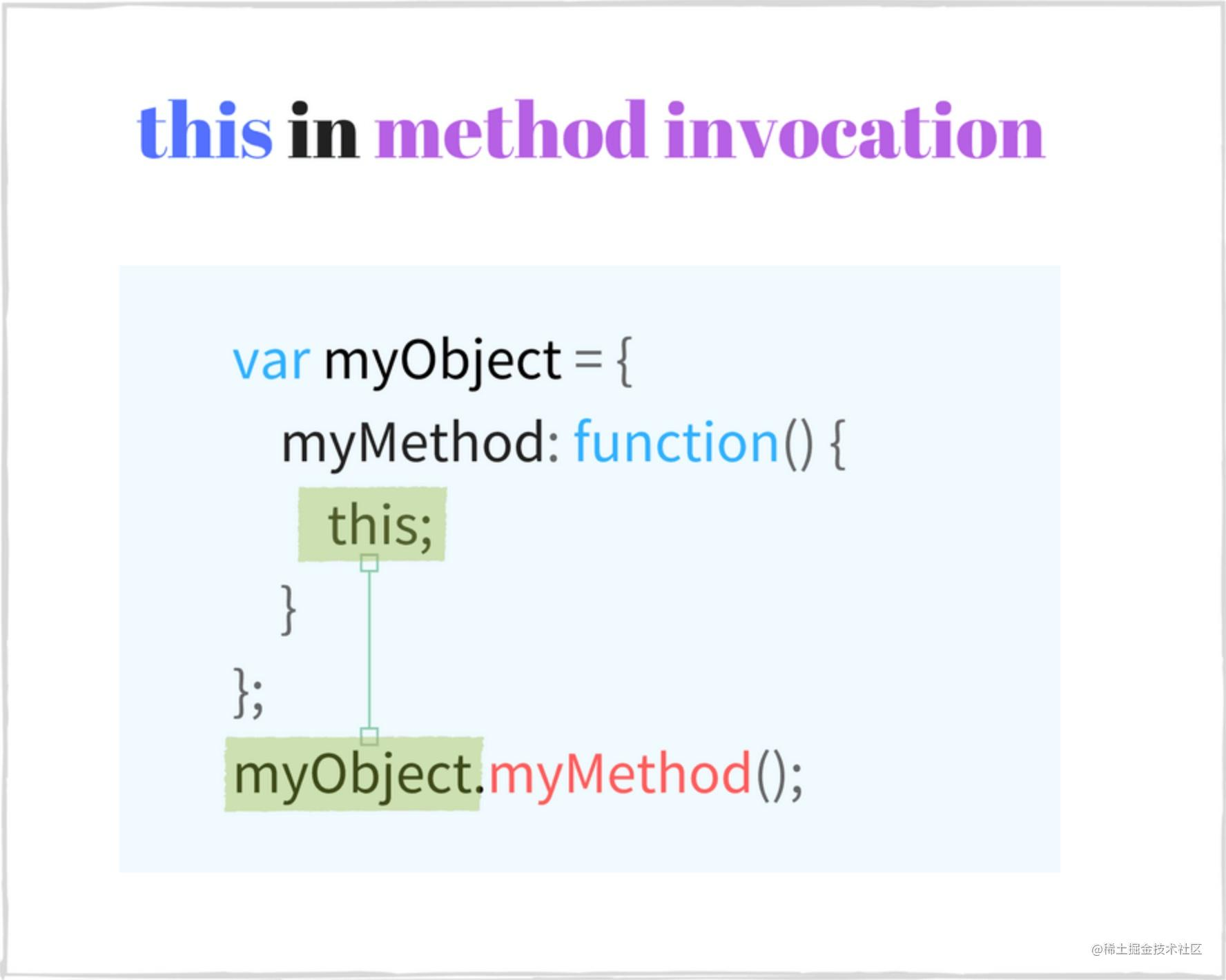const calc = {
num: 0,
increment() {
console.log(this === calc); // => true
this.num += 1;
return this.num;
}
};
// method invocation. this is calc
calc.increment(); // => 1
calc.increment(); // => 2

const myDog = Object.create({
sayName() {
console.log(this === myDog); // => true
return this.name;
}
});
myDog.name = 'Milo';
// method invocation. this is myDog
myDog.sayName(); // => 'Milo'

ECMAScript 2015 中的 class 关键词里，方法调用中的上下文，也就是 this 同样指向它本身。

class Planet {
constructor(name) {
this.name = name;
}
getName() {
console.log(this === earth); // => true
return this.name;
}
}
const earth = new Planet('Earth');
// method invocation. the context is earth
earth.getName(); // => 'Earth'

2.2 陷阱：将对象中的方法抽离

⚠️ 我们可以将对象中的方法提取出来做为一个独立的变量 const alone = myObj.myMethod。当调用函数的时候 alone()，由于它是从原来对象拆出来的，所以可能会认为函数中的 this 会指向 myObject

👍 然而，如果一个方法没有经过对象的属性访问器调用，那它就属于第一节介绍的「函数直接调用」类型，这里的 this 会指向全局对象 window 或者严格模式下的 undefined

function Pet(type, legs) {
this.type = type;
this.legs = legs;
console.log(this === myCat); // => false
console.log(`The \${this.type} has \${this.legs} legs`);
}
}
const myCat = new Pet('Cat', 4);
// logs "The undefined has undefined legs"
// or throws a TypeError in strict mode

// is equivalent to:

👍 同样的，这种情况下我们可以使用 bind 来修复。

function Pet(type, legs) {
this.type = type;
this.legs = legs;
console.log(this === myCat); // => true
console.log(`The \${this.type} has \${this.legs} legs`);
};
}
const myCat = new Pet('Cat', 4);
// Create a bound function
// logs "The Cat has 4 legs"

function Pet(type, legs) {
this.type = type;
this.legs = legs;
console.log(this === myCat); // => true
console.log(`The \${this.type} has \${this.legs} legs`);
};
}
const myCat = new Pet('Cat', 4);
// logs "The Cat has 4 legs"

class Pet {
constructor(type, legs) {
this.type = type;
this.legs = legs;
}
console.log(this === myCat); // => true
console.log(`The \${this.type} has \${this.legs} legs`);
}
}
const myCat = new Pet('Cat', 4);
// logs "The Cat has 4 legs"

3. 构造函数调用

function Country(name, traveled) {
this.name = name ? name : 'United Kingdom';
this.traveled = Boolean(traveled); // transform to a boolean
}
Country.prototype.travel = function() {
this.traveled = true;
};
// Constructor invocation
const france = new Country('France', false);
// Constructor invocation
const unitedKingdom = new Country;
france.travel(); // Travel to France

new Country('France', false) 这种调用方式会创建一个新对象，它的 name 属性是 France

ECMAScript 2015 开始，Js 允许我们通过 class 关键词来定义构造函数。

class City {
constructor(name, traveled) {
this.name = name;
this.traveled = false;
}
travel() {
this.traveled = true;
}
}
// Constructor invocation
const paris = new City('Paris', false);
paris.travel();

new City('Paris') 就是「构造函数调用」类型。创建的对象通过类中一个特殊的方法 constructor 来初始化，其中 this 就指向当前创建的新对象。

3.1 构造函数调用中的 this 指向

this 指向通过构造函数创建的新对象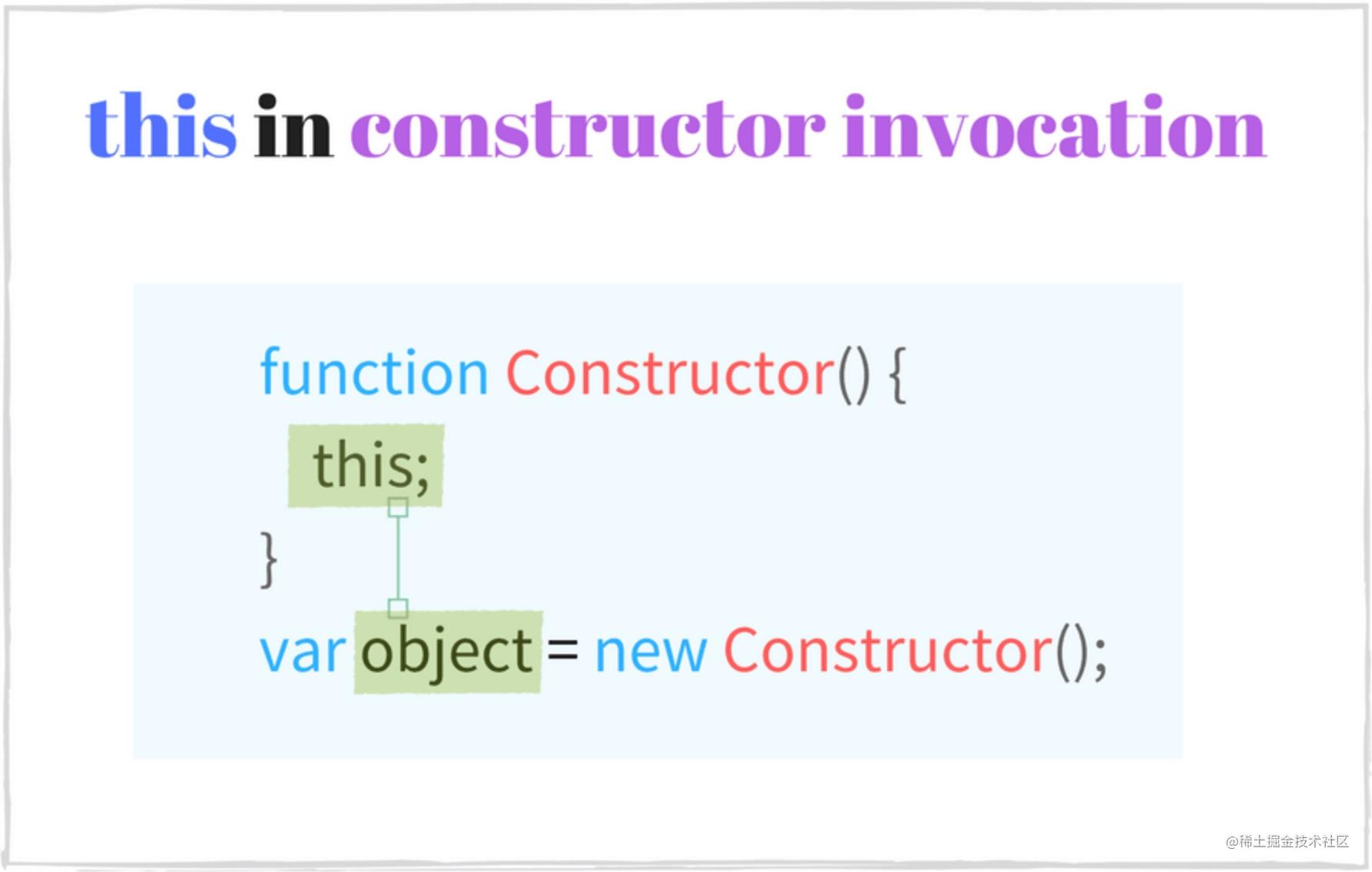function Foo () {
// this is fooInstance
this.property = 'Default Value';
}
// Constructor invocation
const fooInstance = new Foo();
fooInstance.property; // => 'Default Value'

new Foo() 通过构造函数的方式调用，其中的 this 就指向当前的对象 fooInstancethis.property 被初始化了一个值。

class Bar {
constructor() {
// this is barInstance
this.property = 'Default Value';
}
}
// Constructor invocation
const barInstance = new Bar();
barInstance.property; // => 'Default Value'

3.2 陷阱：忘记使用 new

const reg1 = new RegExp('\\w+');
const reg2 = RegExp('\\w+');
reg1 instanceof RegExp; // => true
reg2 instanceof RegExp; // => true
reg1.source === reg2.source; // => true

⚠️ 通过函数直接调用的方式去创建对象可能会有潜在风险，因为如果不使用 new 关键词 一些构造函数可能会忽略掉属性的初始化。

function Vehicle(type, wheelsCount) {
this.type = type;
this.wheelsCount = wheelsCount;
return this;
}
// Function invocation
const car = Vehicle('Car', 4);
car.type; // => 'Car'
car.wheelsCount // => 4
car === window // => true

Vehicle 在上下文对象中会初始化 typewheelsCount 属性。当执行 Vehicle('Car', 4) 的时候将 car 对象返回，拥有正确的属性 car.typecar.wheelCount

👍 为了确保在使用构造函数的时候加上了 new 关键词，我们可以做一些改进：

function Vehicle(type, wheelsCount) {
if (!(this instanceof Vehicle)) {
throw Error('Error: Incorrect invocation');
}
this.type = type;
this.wheelsCount = wheelsCount;
return this;
}
// Constructor invocation
const car = new Vehicle('Car', 4);
car.type               // => 'Car'
car.wheelsCount        // => 4
car instanceof Vehicle // => true
// Function invocation. Throws an error.
const brokenCar = Vehicle('Broken Car', 3);

4. 间接调用

this 指向 .call() 或者 .apply() 所传的第一个参数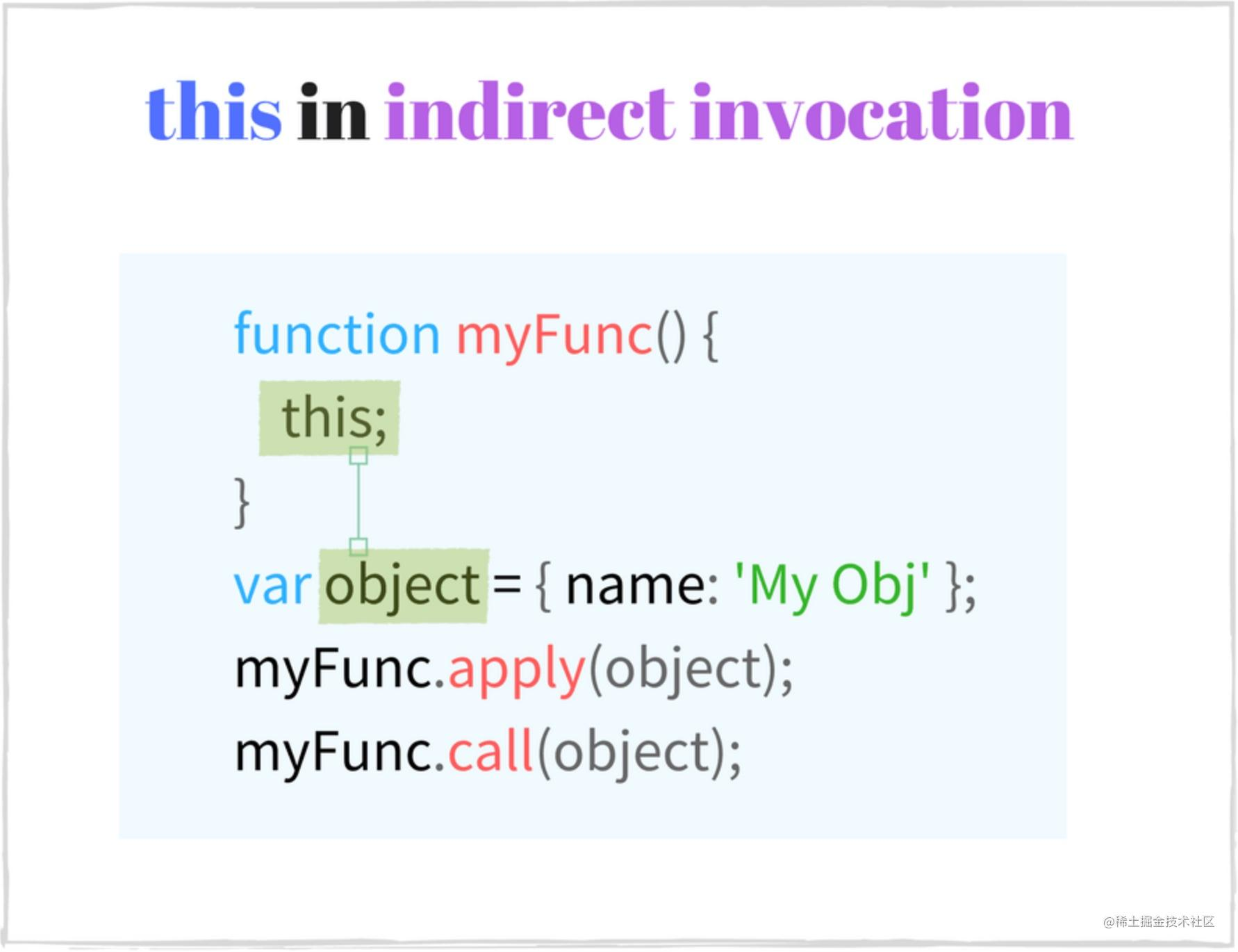const rabbit = { name: 'White Rabbit' };
function concatName(string) {
console.log(this === rabbit); // => true
return string + this.name;
}
// Indirect invocations
concatName.call(rabbit, 'Hello ');  // => 'Hello White Rabbit'
concatName.apply(rabbit, ['Bye ']); // => 'Bye White Rabbit'

function Runner(name) {
console.log(this instanceof Rabbit); // => true
this.name = name;
}
function Rabbit(name, countLegs) {
console.log(this instanceof Rabbit); // => true
// Indirect invocation. Call parent constructor.
Runner.call(this, name);
this.countLegs = countLegs;
}
const myRabbit = new Rabbit('White Rabbit', 4);
myRabbit; // { name: 'White Rabbit', countLegs: 4 }

Rabbit 中调用 Runner.call(this, name) 使得在父函数中的 this 是当前子类相应的对象。

5. 绑定函数

myFunc.bind(thisArg[, arg1, arg2, ...) 的第一个参数 thisArg 作为执行上下文，后边的 arg1, arg2, ... 是要预置的参数值。

function multiply(number) {
'use strict';
return this * number;
}
// create a bound function with context
const double = multiply.bind(2);
// invoke the bound function
double(3); // => 6
double(10); // => 20

multiply.bind(2) 返回了一个新函数 double，相当于 2.multiply

.bind().apply().call() 最大的不同就是，.bind() 是返回一个拥有预设的 this 和参数的新函数，后边还需要被调用才会执行。而 .apply().call() 是调用的时候就执行了。

5.1 绑定函数中的 this 指向

.bind() 函数的作用就是创建一个新的函数，我们需要将要绑定的上下文环境作为第一个参数传给它。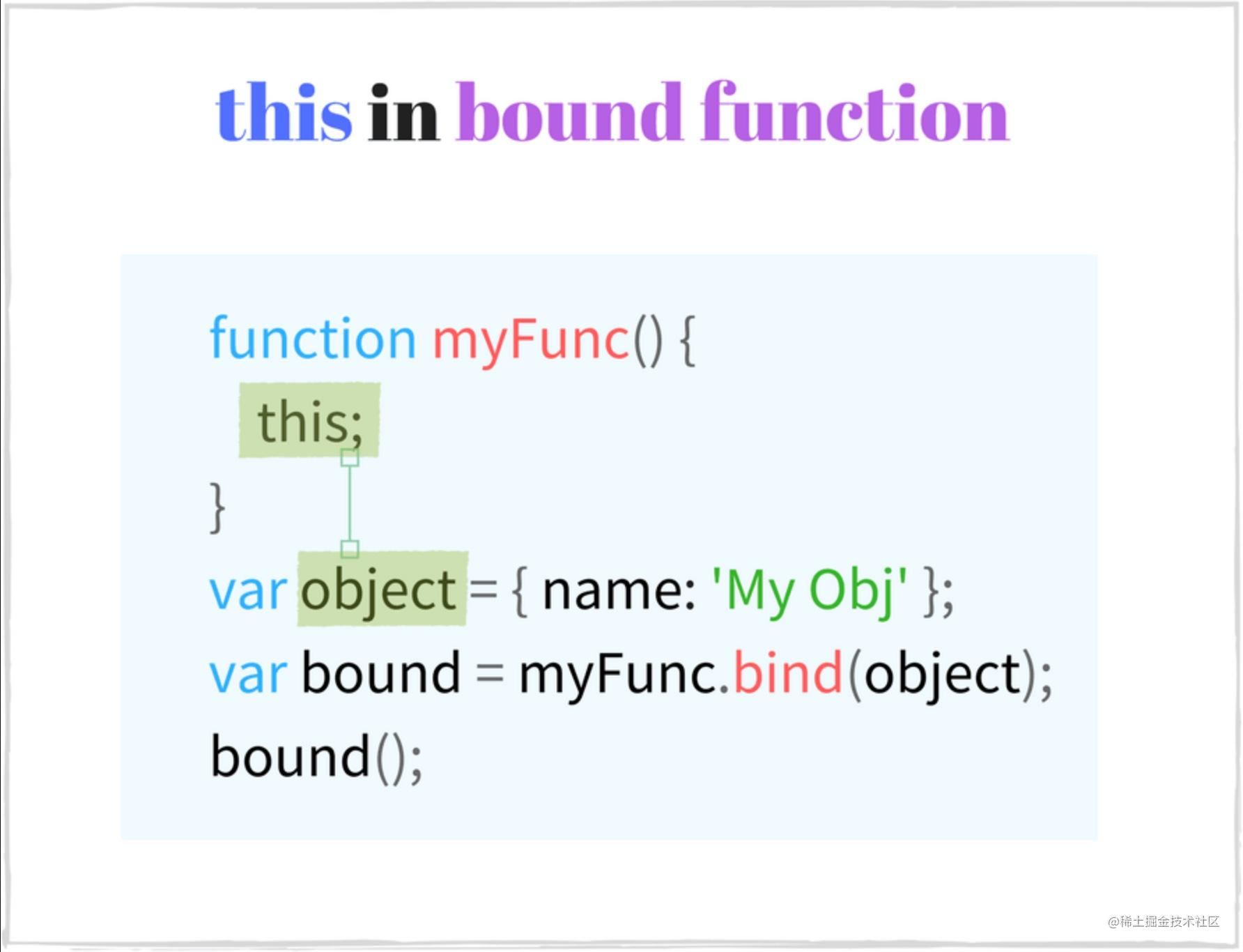const numbers = {
array: [3, 5, 10],
getNumbers() {
return this.array;
}
};
// Create a bound function
const boundGetNumbers = numbers.getNumbers.bind(numbers);
boundGetNumbers(); // => [3, 5, 10]
// Extract method from object
const simpleGetNumbers = numbers.getNumbers;
simpleGetNumbers(); // => undefined or throws an error in strict mode

5.2 牢固的上下文绑定

.bind() 会创造一个永久的上下文绑定，其中的 this 指向不会再发生改变。我们无法再通过 .call().apply() 或者 .bind() 来改变 this 指向。

function getThis() {
'use strict';
return this;
}
const one = getThis.bind(1);
one();         // => 1
one.call(2);   // => 1
one.apply(2);  // => 1
one.bind(2)(); // => 1
new one();     // => Object

6 箭头函数

const hello = (name) => {
return 'Hello ' + name;
};
hello('World'); // => 'Hello World'
// Keep only even numbers
[1, 2, 5, 6].filter(item => item % 2 === 0); // => [2, 6]

6.1 箭头函数中的 this

this 指向定义箭头函数位置的上下文。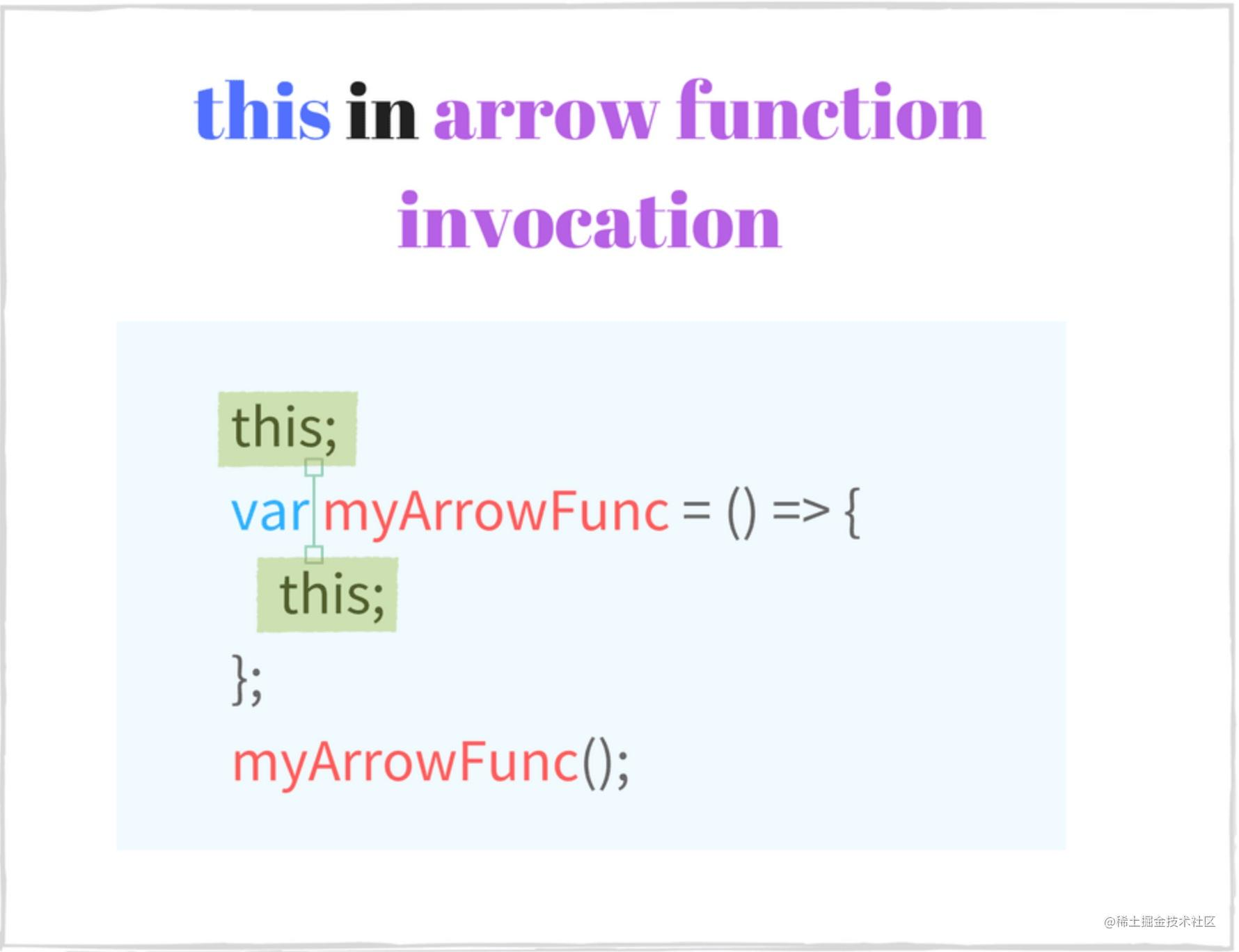class Point {
constructor(x, y) {
this.x = x;
this.y = y;
}
log() {
console.log(this === myPoint); // => true
setTimeout(() => {
console.log(this === myPoint);      // => true
console.log(this.x + ':' + this.y); // => '95:165'
}, 1000);
}
}
const myPoint = new Point(95, 165);
myPoint.log();

setTimeout() 调用箭头函数的时候，它的 this 会指向外部的执行上下文，也就是 myPoint

const getContext = () => {
console.log(this === window); // => true
return this;
};
console.log(getContext() === window); // => true

const numbers = [1, 2];
(function() {
const get = () => {
console.log(this === numbers); // => true
return this;
};

console.log(this === numbers); // => true
get(); // => [1, 2]

// Try to change arrow function context manually
get.call();  // => [1, 2]
get.apply(); // => [1, 2]

get.bind()(); // => [1, 2]
}).call(numbers);

6.2 陷阱：用箭头函数作为一个对象方法

⚠️ 既然箭头函数这么简洁方便，你可能想将对象的一个方法来用箭头函数声明，(param) => {...} 来代替 function(param) {..}

function Period (hours, minutes) {
this.hours = hours;
this.minutes = minutes;
}
Period.prototype.format = () => {
console.log(this === window); // => true
return this.hours + ' hours and ' + this.minutes + ' minutes';
};
const walkPeriod = new Period(2, 30);
walkPeriod.format(); // => 'undefined hours and undefined minutes'

function Period (hours, minutes) {
this.hours = hours;
this.minutes = minutes;
}
Period.prototype.format = function() {
console.log(this === walkPeriod); // => true
return this.hours + ' hours and ' + this.minutes + ' minutes';
};
const walkPeriod = new Period(2, 30);
walkPeriod.format(); // => '2 hours and 30 minutes'

walkPeriod.format() 属于第 2 节介绍「方法调用」类型，所以 this 会指向调用它的对象 walkPeriod，最终也会打印正确的结果。

总结

「函数直接调用」？「方法调用」？「构造函数调用」？「间接调用」？